# B4J Library[B4X] [XUI] xGraph Class and b4xlib

Current version 1.6

The xGraph Class and B4XLib allows graphic analysis of signals.
It works on all three products: B4A, B4i and B4J.

The graph holds a given number of curves (10 by default) with a given number of samples (10000 by default) these values can be defined in the Designer.
All curves have the same number of samples with the same X signal.

What can be done:
- Draw up to 4 curves on the same graph.
- Show values when moving the cursor on the graph.
- Zooming, either with the cursor or setting in the code.
- Different scale modes.
- Different methods on curves.
-- Copy a curve to another location.
-- Add a value to a curve.
-- Multiply a curve by a value.
-- Add two curves and save the result in another curve.
-- Multiply two curves and save the result in another curve.
-- Calculate the integral of a curve and save the result in another curve.
-- Calculate the derivative of a curve and save the result in another curve.

Attached the xGraphDemo.zip file contains three demo program using the xGraph.b4xlib library,
the xGraph.b4xlib library file, the xGraph.bas file and the xGraph.xml file.

The xGraph library needs the XUI, iXUI, or jXUI libraries.
The xGraphDemo programs need the XUI Views.b4xlib library.

EDIT: Version 1.6 2021.09.11
Amended some bugs

EDIT: Version 1.5 2021.03.19
Amended problem with tooltip label remaining visible.

EDIT: Version 1.4 2021.02.22
Set CurveStrokeWidth to Public
Added CalcRMSValue, RMS calculation of a curve
Added CalcSmooth, smoothing of a curve
Added DrawCurve(Index), draws one curve with the given index
Added DrawCurves(Index()), superimposes up to 4 curves with the given indexes
Added SetGraphToSynchronize method, allows to synchronize two xGraph objects.

EDIT: 2020.06.23 Version 1.3
Updated the Tag property according to Erels recommandation:
Added two cursors with display of the curvevalues.
Added a Touch event which return
Added a CursorPositionChanged event returning the CursoorIndex and XIndex.
Added these properties XIndexBegin, XIndexEnd. GraphLeft, GraphTop, GraphWidth and GraphHeight
Version 1.2 2020.02.23
Set CurveColor to Public

EDIT: 2019.12.14 Version 1.1
Amended bug reported HERE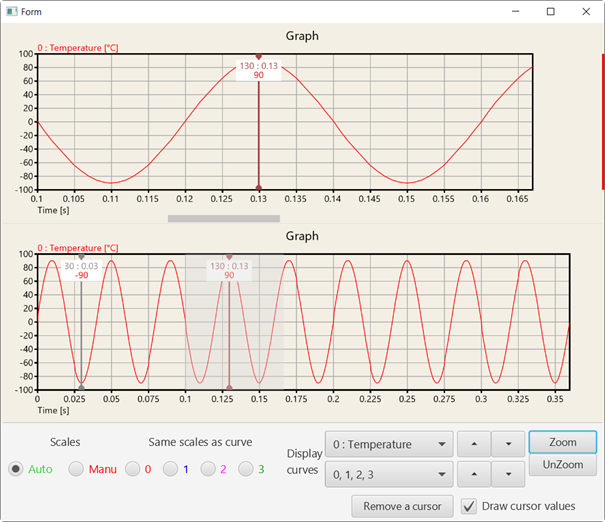Two synchronized graphs, the upper one zoomed, the bottom one not zoomed.
You see the zoomed part of the upper graph in the bottom one, light greaed.

xGraph

Author:
Klaus CHRISTL (klaus)
Version: 1.6
• xGraph
• Events
• CursorPositionChanged (CursorIndex As Int, XIndex As Int)
• Touch (Parameters() As TouchParameters)
TouchParameters (Action As Int, X As Int, Y As Int, XIndex As Int)
X and Y are screen coordinates.
• Methods:
Adds a cursor with the given color by touching the graph
• CalcAddValue (CurveIndex As Int, Value As Double, ZoomedPartOnly As Boolean)
Adds a value to the given cuve
ZoomedPartOnly = True calculates only the zoomed part if the curve is zoomed
• CalcDerivative (SourceIndex As Int, DestinationIndex As Int, ZoomedPartOnly As Boolean)
Calculates the derivative of the source curve and copies the result in the destination curve
ZoomedPartOnly = True calculates only the zoomed part if the curve is zoomed
• CalcDevide2Curves (SourceIndex1 As Int, SourceIndex2 As Int, DestinationIndex As Int, ZoomedPartOnly As Boolean)
Devides two curves and copies the result in the destination curve
Curve(DestinationIndex) = Curve(SourceIndex1) / Curve(SourceIndex2)
ZoomedPartOnly = True calculates only the zoomed part if the curve is zoomed
• CalcIntegral (SourceIndex As Int, DestinationIndex As Int, InitilalCondition As Double, ZoomedPartOnly As Boolean)
Calculates the integral of the source curve and copies the result in the destination curve
ZoomedPartOnly = True calculates only the zoomed part if the curve is zoomed
• CalcMeanValue (CurveIndex As Int, ZoomedPartOnly As Boolean) As Double
Returns the mean value of the given curve
ZoomedPartOnly = True calculates only the zoomed part if the curve is zoomed
• CalcMultiplyByValue (CurveIndex As Int, Value As Double, ZoomedPartOnly As Boolean)
Multiplies the curve by the given value
ZoomedPartOnly = True calculates only the zoomed part if the curve is zoomed
• CalcMutiply2Curves (SourceIndex1 As Int, SourceIndex2 As Int, DestinationIndex As Int, ZoomedPartOnly As Boolean)
Multiplies two curves and copies the result in the destination curve
Curve(DestinationIndex) = Curve(SourceIndex1) * Curve(SourceIndex2)
ZoomedPartOnly = True calculates only the zoomed part if the curve is zoomed
• CalcRMSValue (CurveIndex As Int, ZoomedPartOnly As Boolean)
Returns the RMS value of the given curve.
ZoomedPartOnly = True calculates only the zoomed part if the curve is zoomed.
• CalcSmooth (SourceIndex As Int, DestinationIndex As Int, Span As Int, ZoomedPartOnly As Boolean, SmoothType As String)
Smooths the source curve and saves the result in the destination curve.
The Smooth method takes the mean value of the number of samples given in Span around a sample and saves it at the same index.
Number of samples must be an odd number, if an even number is enterd its value is incremented by 1.
Documentation: https://en.wikipedia.org/wiki/Moving_average.
ZoomedPartOnly = True calculates only the zoomed part if the curve is zoomed.
SmoothType = "MEAN" or "RMS".
Be aware that with RMS smothing, and nagative values, you will get wrong data.
Example code:

xGraph1.CalcSmooth(2, 4, 5, False, "MEAN")
• CalcSmoothExponentialLag (SourceIndex As Int, DestinationIndex As Int, Kt As Double, ZoomedPartOnly As Boolean)
Smooths the source curve and saves the result in the destination curve, sort of low pass filter.
The smoothing algorithm is a first order lag which implements an exponential response to a step input Kt must be smaller than 1.0
yd[t] = yd[t-1] + Kt (ys[t] - yd[t-1])
yd = destination ys = source
Documentation:
https://en.wikipedia.org/wiki/Exponential_smoothing
Routine kindly provided by rgarnett
Example code:

xGraph1.CalcSmoothExponentialLag(2, 4, 0.5, False)
• CalcSmoothExponentialLead (SourceIndex As Int, DestinationIndex As Int, Kt As Double, ZoomedPartOnly As Boolean)
Smooths the source curve and saves the result in the destination curve, sort of high pass filter.
The smoothing algorithm is a first order lead which implements an step response and exponential decay to a step input. Kt must be smaller than 1.0.
yd = Kt (ys[t]- yd[t-1])
ydLast = y[t-1] + yt
yd = destination ys = source.
Routine kindly provided by rgarnett1955.
Example code:

• CalcSubtract2Curves (SourceIndex1 As Int, SourceIndex2 As Int, DestinationIndex As Int, ZoomedPartOnly As Boolean)
Substracts two curves and copies the result in the destination curve
Curve(DestinationIndex) = Curve(SourceIndex1) - Curve(SourceIndex2)
ZoomedPartOnly = True calculates only the zoomed part if the curve is zoomed
• CalcSum2Curves (SourceIndex1 As Int, SourceIndex2 As Int, DestinationIndex As Int, ZoomedPartOnly As Boolean)
Adds two curves and copies the result in the destination curve
Curve(DestinationIndex) = Curve(SourceIndex1) + Curve(SourceIndex2)
ZoomedPartOnly = True calculates only the zoomed part if the curve is zoomed
• CopyCurve (SourceIndex As Int, DestinationIndex As Int)
Copies the curve with SourceIndex to the DestinationIndex curve.
• DeSynchronize
Desynchronizes two synchronized xGraph objects.
• DrawCurve (Index As Int)
Draws the curve with the given index.
Example code:

xGraph1.DrawCurve(2)
• DrawCurves (Indexes() As Int)
Draws the curves with the given indexes.
Example code:

xGraph1.DrawCurves(Array As Int(0,1,2,3))
• DrawGraph
Draws a graph
• GetScaleAuto (CurveIndex As Int) As ScaleValues
Gets the automatic scale for the given curve
• GetScaleManu (CurveIndex As Int) As ScaleValues
Gets the manual scale for the given curve
• Initialize (Callback As Object, EventName As String)
• NumberFormat3 (Number As Double, MaxDigits As Int) As String
Formats numbers with a fixed number of digits and scientific notation
• RemoveCursor (Index As Int)
Removes one or both cursors.
Set 0 or 1 as cursor index to delete one cursor.
Set 2 to delete both cursors.
When you remove cursor(0) cursor(1) becomes cursor(0), it is removed from the list.
If there is only one Cursor, then any index value will remove the cursor.
• SelectGraph (Selected As Boolean)
Sets the xGraph object to Selected or not.
Selected = True, draws a colored line at the right side.
Selected = False, removes the colored line.
Used when two xGraph objects are synchronized.
Draws a line at the right side of the graph.
• SetCursor (XIndex As Int, CursorIndex As Int, Color As Int)
Sets one of the two cursors.
XIndex = sample index, in the curve array.
CursorIndex = 0 or 1, index of the cursor.
• SetCurveName (CurveIndex As Int, Name As String, UpdateGraph As Boolean)
Sets the a new name for the curve with the given index
UpdateGraph = True redraws the graph
• SetCurveUnit (CurveIndex As Int, Unit As String, UpdateGraph As Boolean)
Sets a new unit for the curve with the given index
UpdateGraph = True redraws the graph
• SetGraphToSynchronize (GraphToSynchronize As XGraph, SelectedGraphShow As Boolean, SelectedGraphColor As Int, SynchronizeZoom As Boolean, DisplaySynchronizedZoomArea As Boolean)
If you have two xGraph objects you can synchronize them.
Condition: the X scales must be the same.
Cursors and zoom are active on both objects.
GraphToSynchronize = xGraph object to synchronize.
SelectedGraphShow = True shows a line at the right side of the graph, with the SelectedGraphColor.
SelectedGraphColor = color of the line at the right side of the graph.
SynchronizeZoom = True synchronizes the zoom in both graphs.
= False you can have two different zoom levels in the two graphs.
DisplaySynchronizedZoomArea = True, when one graph is zoomed and the other not, displays the zoomed area in the other graph.
= False, does not display the zoomed area.
Example code:

xGraph1.SetGraphToSynchronize(xGraph2, True, xui.Color_Red, True)
xGraph2.SetGraphToSynchronize(xGraph1, True, xui.Color_Blue, True)

To synchronize two xGraph objects, you need to add the two lines above in your code.
• SetSameScale (SameScaleCurveIndex As Int)
Sets the scale of curve SameScaleCurveIndex for all curves
• SetScaleManu (CurveIndex As Int, MinVal As Double, MaxVal As Double)
Sets a manual scale for a given curve
• SetZoomIndexes (BeginIndex As Int, EndIndex As Int)
Sets the begin and end indexes for zoom diaplay
• SetZoomMode
Sets the zooming mode
The user selects with the curor the left limit and then the right limit
• UnZoom
• Properties:
• AutomaticYScales
Gets or sets the automatic scales mode
True displays automatic Y sacales for all curves
False displays the manual scales
• CurvesToDisplay
Gets or sets the curves to display
• DisplayCurveIndex
Gets or sets the DisplayCurveIndex property
True displays the curve index before the curve name
Example: 3 : Speed
• DisplayCurveUnit
Gets or sets the DisplayCurveUnit property
True displays the curve unit after the curve name
Example: Speed [m/s]
• DisplaySynchronizedZoomArea
When two xGraph objects are synchronized and when one graph is zoomed and the other not,
True > displays the zoomed area in the other graph
False > does not display the zoomed area in the other graph.
• DrawCursorValues
gets or sets the DrawCursorValues property
Displays the values of the cuves at the cursor position
• GraphColor
Gets or sets the graph background color
• GraphHeight
Gets the GraphHeight property
The height of the Graph in pixels
• GraphLeft
Gets the GraphLeft property
The left position of the Graph in pixels
• GraphTop
Gets the GraphTop property
The top position of the Graph in pixels
• GraphWidth
Gets the GraphWidth property
The width of the Graph in pixels
• GraphWithMissingData
Gets or sets the GraphWithMissingDat property
Manages curves with missing data, it is necessary to enter the MissingDataValue in place.
• GridColor
Gets or sets the graph grid color
• GridFrameColor
Gets or sets the graph grid frame color
• Height
Gets or sets the Height property
• Left
Gets or sets the Left property
• MissingDataValue
Gets or sets the MissingDataValue property
Used when GraphWithMissingData = True, the missing data must be replaced by this value
• NbMaxCurves
Gets or sets the max number of curves
• NbMaxSamples
Gets or sets the max number of samples
• NbSamples
Gets or sets the current number of samples
• NbXIntervals
Gets or sets the number of x axis intervals
• NbYIntervals
Gets or sets the number of y axis intervals
• NumberOfCursors
Gets the current number of cursors.
• OuterFrame
Gets or sets the graph outer frame property
True = draws an outer frame around the graph
• OuterFrameColor
Gets or sets the graph outer frame color
• ScaleXValues
Gets or sets the ScaleValues property
it is a string with the different scale values separated by an exclamation mark.
it must begin with 1! and end with !10
Example: the default property 1!2!2.5!5!10
These values can be selected in the Designer: 1!2!2.5!5!10|1!1.2!1.5!1.8!2!2.5!3!4!5!6!7!8!9!10
• ScaleYValues
Gets or sets the ScaleValues property
used for automatic scales
it is a string with the different scale values separated by the exclamation mark.
it must begin with 1! and end with !10
Example: the default property 1!2!2.5!5!10
These values can be selected in the Designer: 1!2!2.5!5!10|1!1.2!1.5!1.8!2!2.5!3!4!5!6!7!8!9!10
• Snapshot
Returns a B4XBitmap object of the graph (read only)
• SynchronizeZoom
When two xGraph objects are synchronized.
Synchronize the zoom in both graphs.
• Title
Gets or sets the graph title
• Top
Gets or sets the Top property
• UseCustomColors
Gets or sets the UseCustomColors property.
UseCustomColors = False, 4 colors and widths are used for superimpositions they remain the same independant of the curves displayed.
UseCustomColors = True, one specific color and width can be defined for each curve.
• Width
Gets or sets the Width property
• XAxisName
Gets or sets the x axis name
• XAxisUnit
Gets or sets the x axis uni
• XIndexBegin
Gets the XIndexBegin property
Index of the first visible item
• XIndexEnd
Gets the XIndexBegin property
Index of the last visible item

#### Attachments

• xGraphDemoV1_6.zip
198.3 KB · Views: 17
• xGraph.b4xlib
16.9 KB · Views: 18
• xGraph.bas
94.4 KB · Views: 19
• xGraph.xml
34.3 KB · Views: 17
Last edited:

#### budakbaru

##### Member
Yes I expect 1 peak also but different amplitude. I just want to test whether CalcIntegral method can be use for FFT Magnitude signal or not.
The x axis value I just make it. Also for CalcRMSValue, can it be used for FFT Amplitude signal to get the same or almost same value as original sine wave rms.

Thanks.

#### klaus

##### Expert
In the mean time, I played a little bit with your project.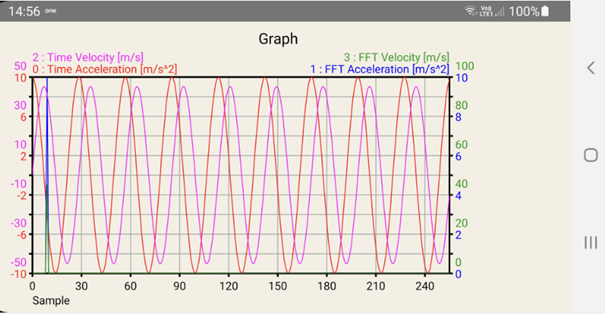If you have an integer number of sine cycles in the time window you get one peak.
The amplitude returned by the FFT calculation is the sine amplitude.

I just want to test whether CalcIntegral method can be use for FFT Magnitude signal or not.
What exactly do you mean with this ?

#### Attachments

• Xgraph2.zip
25.9 KB · Views: 60
Last edited:
•Erel

#### budakbaru

##### Member
Thanks klaus.
I'm not very good in calculus but I like to learn it. I thought it is ok just to use calcintegral for FFT magnitude.

B4X:
``````For i = 0 To Samples - 1
TimeVelocity(i) = xGraph1.CurveY(TimeVelIndex, i)
Next``````
this is the secret.

Many thanks Klaus.

#### klaus

##### Expert
The xGraph library has been updated to version 1.4.

Set CurveStrokeWidth to Public
Added CalcRMSValue, RMS calculation of a curve
Added CalcSmooth, smoothing of a curve
Added DrawCurve(Index), draws one curve with the given index
Added DrawCurves(Index()), superimposes up to 4 curves with the given indexes
Added SetGraphToSynchronize method, allows to synchronize two xGraph objects.

•#### budakbaru

##### Member
Hai Klaus.

Thanks again for you excellent graph plotting.

I would like to know how to clear the label when cursor added or zoom function selected. I mean after the cursor added to the graph then the label disappear.

Thank a lot

#### budakbaru

##### Member
Dear Klaus,

How to plot 2 curves with different number of index but same scale as below.

Curve 1
index valueX valueY
0 0 0
1 0.1 1
2 0.2 2
3 0.3 1
4 0.4 0
5 0.5 -1
6 0.6 -2
7 0.7 -1
8 0.8 0
9 0.9 1
10 1.0 2

Curve 2
index valueX valueY
0 0 1
1 0.2 0
2 0.4 -1
3 0.6 0
4 0.8 1
5 1.0 0

Curve 1 has many samples than curve 2 but final value of X still same.

#### klaus

##### Expert
I suppose that you are speaking of the tooltip label on top of the graph.
Can you please explain more in detail what you are doing when it happens.
It probably happens when you have two synchronized graphs and you have selected one, activate the zoom function but move the cursor on the other graph.
You need to finish the method in the selected graph.

How to plot 2 curves with different number of index but same scale as below.
You cannot. The data structure is not made for this.
You can do it with a YXChart, there the data structure is different.

#### budakbaru

##### Member
I suppose that you are speaking of the tooltip label on top of the graph.
Can you please explain more in detail what you are doing when it happens.
It probably happens when you have two synchronized graphs and you have selected one, activate the zoom function but move the cursor on the other graph.
You need to finish the method in the selected graph.

In B4A when I want to set a cursor there will be a label appear say select the position of the cursor. After select on the graph, the label still there.
Same for zoom function. The label stay there until exit the apps.
But for B4J the label will disappear immediately after the position of the cursor on the graph selected.

Thanks.

#### klaus

##### Expert
I see it too.
Thank you for reporting it.
Amended for the next version.
Attached the two files of the current not yet official version 1.5.

EDIT: Removed the two files, they are in the first post.

Last edited:

#### budakbaru

##### Member
Dear Klaus and all,
Can someone tell me why I got 2 peaks in graph for below code. This is not related directly to xgraph. But maybe Klaus now the answer.

B4X:
``````#Region  Project Attributes
#ApplicationLabel: B4A XGraph Integral
#VersionCode: 1
#VersionName:
'SupportedOrientations possible values: unspecified, landscape or portrait.
#SupportedOrientations: unspecified
#CanInstallToExternalStorage: False
#End Region

#Region  Activity Attributes
#FullScreen: False
#IncludeTitle: False
#End Region

Sub Process_Globals
'These global variables will be declared once when the application starts.
'These variables can be accessed from all modules.
Private xui As XUI
Private Samples As Int
End Sub

Sub Globals
'These global variables will be redeclared each time the activity is created.
Private xGraph1 As xGraph
Private FFT As xFFT
Private TimeAccelleration(256), TimeVelocity(256) As Double
Private FFTMagAccelleration(), FFTMagVelocity() As Double
Private SineAmplitude, SineCyclesNumber, Omega As Double
Private TimeAccIndex = 0 As Int
Private TimeVelIndex = 2 As Int
Private FFTAccIndex = 1 As Int
Private FFTVelIndex = 3 As Int
End Sub

Sub Activity_Create(FirstTime As Boolean)
FFT.Initialize

InitGraph
End Sub

Sub Activity_Resume

End Sub

Sub Activity_Pause (UserClosed As Boolean)

End Sub

Private Sub InitGraph
Private i As Int

xGraph1.CurveYName(TimeAccIndex) = "Time Acceleration"
xGraph1.CurveYUnit(TimeAccIndex) = "m/s^2"
xGraph1.CurveYName(TimeVelIndex) = "Time Velocity"
xGraph1.CurveYUnit(TimeVelIndex) = "m/s"
xGraph1.CurveYName(FFTAccIndex) = "FFT Acceleration"
xGraph1.CurveYUnit(FFTAccIndex) = "m/s^2"
xGraph1.CurveYName(3) = "FFT Velocity"
xGraph1.CurveYUnit(3) = "m/s"

xGraph1.CurvesToDisplay.Initialize2(Array As Int(0, 1, 2, 3))

Samples = 256
xGraph1.NbSamples = Samples

' number of sines in the time window (256 samples)
' you can play with NumberOfSines
' setting an integer number gives 1 frequency peak.
' with the values below you can see in the graph that:
' the frequency peak is at 9, which is the SineCyclesNumber
' the amplitude of the peak is 10, which is the SineAmplitude.
' setting a non integer value for the SineCyclesNumber will lower  the amplitude a bit and 'spread' the peak
'    SineCyclesNumber = 9.0
SineCyclesNumber = 100.0
SineAmplitude = 10
Omega = 360 * SineCyclesNumber / Samples
For i = 0 To Samples - 1
TimeAccelleration(i) = SineAmplitude * CosD(i * Omega)
xGraph1.CurveY(TimeAccIndex, i) = TimeAccelleration(i)
xGraph1.CurveX(i) = i
Next

xGraph1.CalcIntegral(TimeAccIndex, TimeVelIndex, 0, False)

For i = 0 To Samples - 1
TimeVelocity(i) = xGraph1.CurveY(TimeVelIndex, i)
Next

'    FFTMagAccelleration = FFT.Forward(TimeAccelleration)
FFTMagVelocity = FFT.Forward(TimeVelocity)

For i = 0 To Samples / 2 - 1
'        xGraph1.CurveY(FFTAccIndex, i) = FFTMagAccelleration(i)
xGraph1.CurveY(FFTVelIndex, i) = FFTMagVelocity(i)
Next

xGraph1.CurveYName(TimeVelIndex) = "Time Velocity"
xGraph1.CurveYUnit(TimeVelIndex) = "m/s"
xGraph1.DrawCurves(Array As Int(FFTVelIndex))
End Sub``````

#### klaus

##### Expert
You need to give more explanation and information on what you want to achieve, what you have done and how !

So, the problem is the numeric integration with a very very low number of data for one period of the sine curve !
You have 256 time samples and 100 sine periods which means that you have 2.5 points per sine period and this is by far not enough.
To get correct results you must have at least 10 points per sine period.

Image of the acceleration with 100 sine periods, zoomed.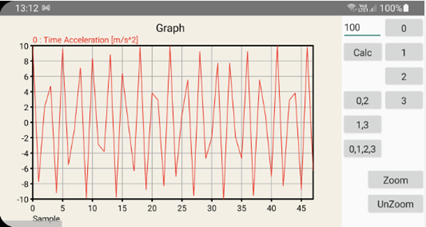This signal looks more like a random signal rather than a pure sine.

Image of the acceleration and the integration, velocity, zoomed.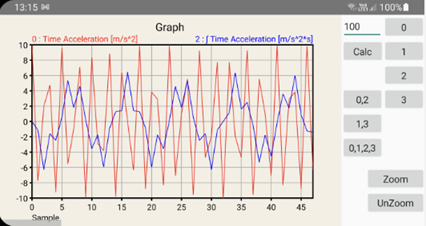Image with 30 sine periods ~10 points per sine period with the same zoom.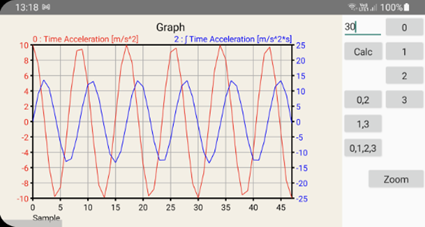And the four signals unzoomed, and here you have only 1 peak as expected !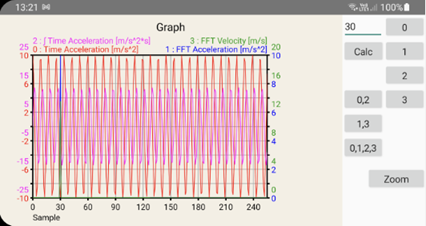Attached the test project.

Now, what exactly do you want to achieve ?
I really do not understand your approach of this subject !?

#### Attachments

• Xgraph3.zip
27.9 KB · Views: 45
Last edited:
•Peter Simpson

#### klaus

##### Expert
The xGraph library has been updated to version 1.5.

Amended problem with the tooltip label remaining displayed, reported in post #45.

•Peter Simpson and Jorge M A

#### budakbaru

##### Member
You need to give more explanation and information on what you want to achieve, what you have done and how !

So, the problem is the numeric integration with a very very low number of data for one period of the sine curve !
You have 256 time samples and 100 sine periods which means that you have 2.5 points per sine period and this is by far not enough.
To get correct results you must have at least 10 points per sine period.

Image of the acceleration with 100 sine periods, zoomed.

View attachment 110035

This signal looks more like a random signal rather than a pure sine.

Image of the acceleration and the integration, velocity, zoomed.

View attachment 110036

Image with 30 sine periods ~10 points per sine period with the same zoom.

View attachment 110037

And the four signals unzoomed, and here you have only 1 peak as expected !

View attachment 110038

Attached the test project.

Now, what exactly do you want to achieve ?
I really do not understand your approach of this subject !?
Thanks Klaus. So I need to increase no of samples. Understand.
I'm reading about FFT conversion and try to see the result using what B4A function and library have.

Thanks for you long explanation and sample program.

#### klaus

##### Expert
The xGraph library has been updated to version 1.6.
Amended some bugs.

•Replies
81
Views
12K
Replies
19
Views
2K
Replies
4
Views
857
Replies
1
Views
951
B4J Code Snippet [B4X] Bezier curve
Replies
2
Views
2K